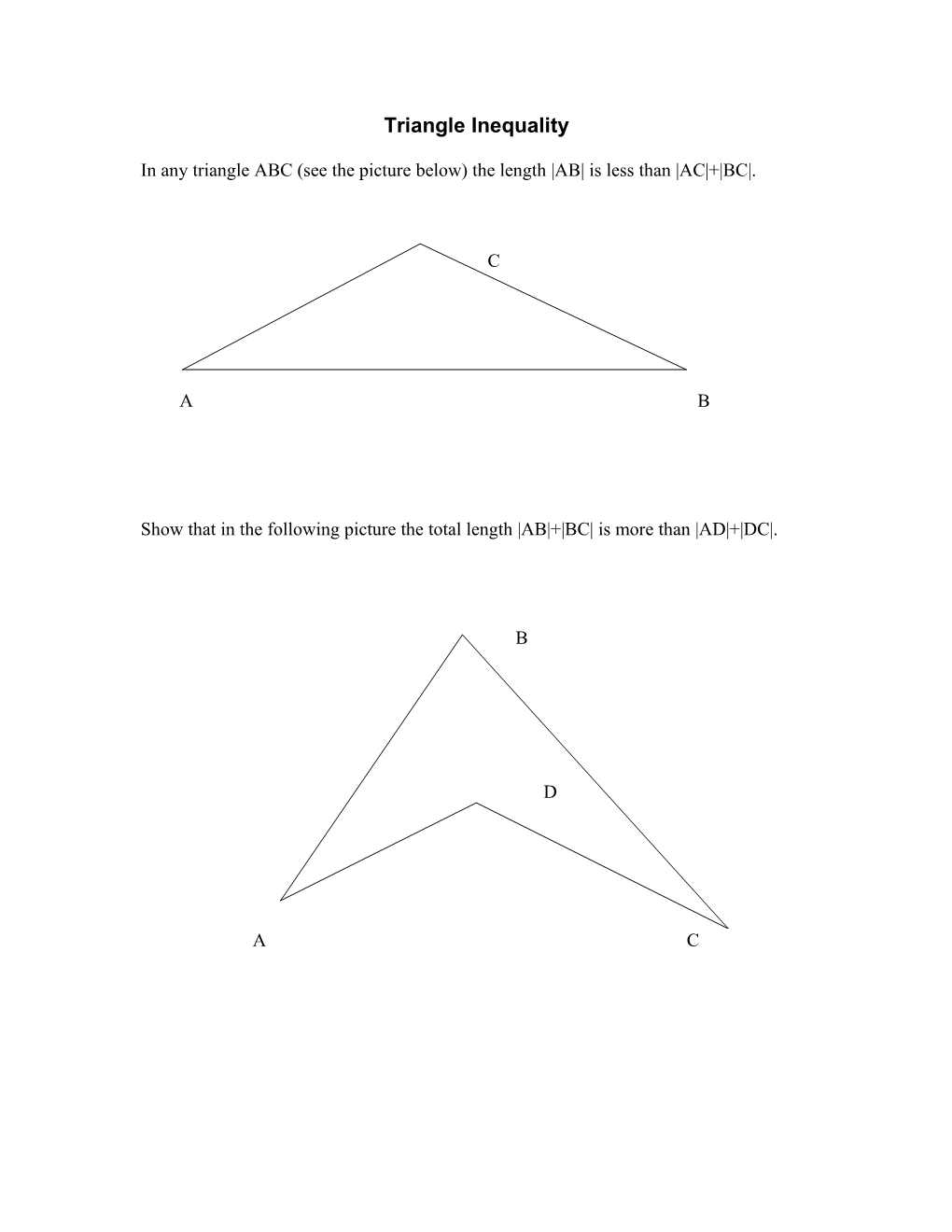# In Any Triangle ABC (See the Picture Below) the Length AB Is Less Than AC + BC#### Triangle Inequality

In any triangle ABC (see the picture below) the length |AB| is less than |AC|+|BC|.

Show that in the following picture the total length |AB|+|BC| is more than |AD|+|DC|.

#### The Area

In the picture below, E is the midpoint of AB and F is the midpoint of AD. If the area of FAEC is 13, what's the area of ABCD?#### The "Middle" Triangle

In rectangle ABCD, E is the midpoint of BD, F is the midpoint of ED, AD is 10 cm, and AB is 20 cm. What's the area of AEF?#### Pentamino

A "net" is a two-dimensional pattern that you could cut out and fold up to get a three-dimensional shape. The net of a cube is made up of six squares, but they must be arranged in certain ways so that the squares won't overlap when it's folded up.

The twelve shapes below are called "pentominoes." You might be familiar with dominoes - the "do-" stands for two, so they're two squares that share an edge. The "pent-" in pentominoes stands for five, so they have five squares that share edges. There are only twelve ways to arrange five squares (two pentominoes are considered the same if they are congruent - if you can flip or rotate one to match it up to another, they're the same).Your first task: Figure out which of the pentominoes above will not work as part of the net of a cube.

Let's look at one that will work. If we take pentomino A above, we could add a blue square to the bottom and fold it up to make a cube (try it!). If you play around with the other eleven pentominoes, you'll find that some of them simply won't work no matter where you add an extra square. So you need to find which ones won't work, and explain how you figured it out.

Your second task: Take three of the ones that will work and explain where to add a sixth square to make it a net of a cube. There will probably be more than one place where you could add a square, so make sure you name them all. Be sure to be clear with your explanation, as it might be hard to understand without a picture.

#### The Perimeter

This is an isosceles triangle. AC and AB are both equal to 5 inches, and angle CAB is greater than or equal to 60 degrees. If the edge lengths of the triangle must all be integers, what's the perimeter of the triangle?

#### Napoleon's River

Napoleon is marching along some flat ground with his troops, and they come to a river. Napoleon wants to know the width of the river before they cross (though if you ask me, I'd be more concerned about the depth!). Anyway, one of the soldiers steps up to the edge of the river and faces directly across the water. He adjusts his cap until the tip of the visor is in line with his eye and the edge of opposite bank. Then he turns around and faces away from the river. He notes the spot on the ground that is now in line with his eye and the tip of his visor, and paces off that distance, announcing that it is equal to the width of the river.

#### Picking Painted Cubes

A large cube is made up of smaller cubes. The edge of the big cube is five little cubes long. All of the faces of this big cube are painted; then the cube is taken apart and the little cubes are put in a bag. You pick a cube out of the bag. What's the probability that the cube you choose has

• three faces painted?
• two faces painted?
• one face painted?
• no faces painted?

Bonus: Make the edgelength of the large cube n. Now what are the answers?

#### Rotating a Triangle

Take a look at the triangle shown below. If you rotate the triangle 90 degrees about the origin, what will be the new coordinates of the vertices?#### Nine Congruent Rectangles

These nine congruent rectangles form a larger rectangle whose area is 720 units2. What's the perimeter of the large rectangle?

Make sure you explain what you did to figure it out!